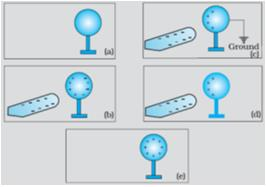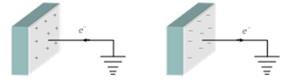Courses

# Test: Electric Charge

## 20 Questions MCQ Test Physics Class 12 | Test: Electric Charge

Description
This mock test of Test: Electric Charge for Class 12 helps you for every Class 12 entrance exam. This contains 20 Multiple Choice Questions for Class 12 Test: Electric Charge (mcq) to study with solutions a complete question bank. The solved questions answers in this Test: Electric Charge quiz give you a good mix of easy questions and tough questions. Class 12 students definitely take this Test: Electric Charge exercise for a better result in the exam. You can find other Test: Electric Charge extra questions, long questions & short questions for Class 12 on EduRev as well by searching above.
QUESTION: 1

### A material in which electrons are tightly bound and cannot move freely at room temperature is called

Solution:

Insulators are those materials in which electron are tightly bound and cannot move freely at room temperature.

QUESTION: 2

### According to the principle of conservation of charge, in closed system

Solution:

The law of conservation of charge states that electric charge can neither be created nor destroyed. In a closed system, the amount of charge remains the same. When something changes its charge it doesn't create charge but transfers it.

QUESTION: 3

### A coulomb is same as:​

Solution:

Current = charge/time

Therefore charge = current. time (unit of current = ampere, unit of time=second) so, charge = ampere.second

QUESTION: 4

When a glass rod is rubbed with silk, it becomes positively charged because

Solution:

The -ve charges of glass rod gets transferred to the silk cloth and hence itself becomes positively charged as there is deficiency of -ve charge(electrons) on the glass rod due to their transfer. But total charge of system of rod and cloth remain zero

QUESTION: 5

Which of the following methods can be used to charge a metal sphere positively without touching it? Choose the best possible option:

Solution:

This can be possible by induction, if a negative charge rod is placed near the sphere (at a distance not in touch) than it attracts positive charge inside the sphere towards its side and the negative charges collected on other side and when the surface of sphere connected to earth than the negative charge goes to earth and the sphere remain positively charged.QUESTION: 6

If mica and woolen cloth are rubbed together, then mica gets

Solution:

Woolen cloth has +ve charge and when mica is rubbed on it , mica gets +vely charged due to (conduction) - flow of charge from wool to mica

QUESTION: 7

Choose the correct statement

Solution:

The theoretical reason for making the conclusion that the total charge of the universe is constant is the Law of Conservation of Charge. Because of certain symmetries in the structure of the universe, the total electric charge of an isolated system is always conserved. This means that the total charge of an isolated system is the same at all points in time.

QUESTION: 8

To make an uncharged object to have a negative charge we must:

Solution:

To make an uncharged object have a negative charge we must increase charge on particle and this can be done only by adding electrons to particle.

QUESTION: 9

When conductors are placed in an electric field, their electrons are moved

Solution:

A substance is said to be conductor if it conduct the electricity .In case of a conductor , the electrons are move in the opposite direction of current ,so it is able to conduct .

QUESTION: 10

When a negatively charged conductor is connected to earth,

Solution:

After earthing a positively charged conductor electrons flow from earth to conductor and if a negatively charged conductor is earthed then electrons flows from conductor to earth.QUESTION: 11

A negative ion is an atom that ________ electrons

Solution:

Ions are formed when atoms gain or lose electrons. Gaining electrons changes an atom into a negative ion. Losing electrons changes an atom into a positive ion.

QUESTION: 12

When a hard rubber rod is given a negative charge by rubbing it with wool:

Solution:

When a hard rubber rod is given a negative charge by rubbing it with wool, negative charges are transferred from wool to rod.

QUESTION: 13

The value of charge on a body which carries 30 excess electrons is

Solution:

Here, n = 30 q = ?
e = -1.6 x 10-19 C
So, q = n ∗ e = 30 (1.6 x 10-19 C)
q = -4.8 ∗ 10-18 C

QUESTION: 14

The total negative charge in 1 mol of helium (atomic number 2, atomic mass 4) is:

Solution:

He atom has 2 electrons. so 1 mole of He has 2*N(N is Avohadro's no.) electrons. then total -ve charge in 1 mole He gas is 2*N*charge of 1 electron. calculating we will get about 1.9 x 105 C.

QUESTION: 15

When we rub a glass rod with silk, then

Solution:

when glass rod is rubbed with silk electrons are transferred from glass to silk, therefore the mass of glass rod decreases

QUESTION: 16

How many electrons are present in 1 coulomb of charge?​

Solution:

According to Quantisation of Charge we know that,

Q = ne

therefore , 1 coloumb of Charge = n . 1.6 × 10-19

=>1/ 1.6 × 10-19 = n

6.25 × 1018 = n

QUESTION: 17

What does q1 + q2 = 0 signify?​

Solution:

If we have two bodies with charge q1 and q2 on them and we are given q1 + q2 = 0. It means the net charge on the system is zero.

This is possible only if q1 and q2 are equal and opposite.

That is, q1 = +q and q2 = -q or vice versa.

Such that, q1 + q2 = +q + (-q) = 0

QUESTION: 18

A silk cloth rubbed with a glass rod acquire a charge (-1.6 x 10-19) C. Then the charge on the glass rod is​

Solution:

During rubbing ,glass rod acquires + ve charges and silk cloth acquires - ve charges.therefore, the charge on the glass rod is + 1.6×10- 19C

QUESTION: 19

To make uncharged object have a positive charge:

Solution:

A body consists of atoms which have electrons, protons and neutrons. The electrons have negative charge. The protons are positively charged and the neutrons are neutral. The atom is neutral because the positive and negative charges cancel out each other. On removing electrons , an excess of protons are present and thus the body is said to be positively charged.

QUESTION: 20

Electric charge between two bodies can be produced by

Solution:

Electric charge between two bodies can be produced by rubbing with each other . The body having lower work function looses electron and becomes positive and the other body gains electrons become negative . Example -- When glass is rubbed with silk , glass becomes positive while silk becomes negative .